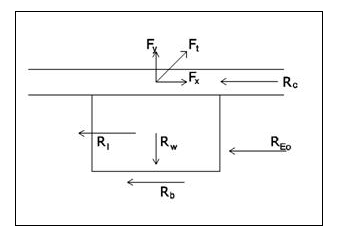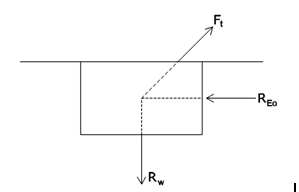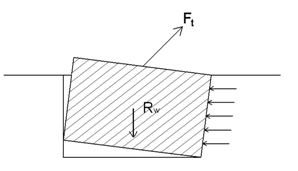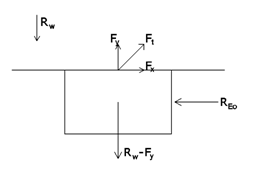##### IntroductionIn this case, pulling force Ft has two components. vertical Fy and horizontal FxFy has already been studied in the previous section (although it will be re-studied later on when considering footing rotation).

Now, we will pay attention to the behaviour of FxIn order to guarantee stability, the footing will put up resistance to Fx in some different ways.

• Re soil lateral resistance, produced by lateral earth pressure E0
• Rf  friction of the footing base Rb and lateral sides Rl against the soil.
• Rf = Rb + Rl
• Rc compression resistance of pavement only if it exists.

Rx = Re + Rf + Rc

Les’s study each of these stabilization factors.

##### Lateral soil resistance

It is the most reliable and important resource to stabilize the horizontal force applied to the footing. In many cases there is no pavement, so Rc = 0 and if the pulling vertical force equals footing weight (we have seen that this is more than probable), footing base friction will be 0 as well, Rb = 0. If we consider the possibility that footing lateral friction Rl is 0 as well due to the rain or soil humidity, we’ll have Rx = Re.

In the previous section we have defined earth pressure at rest value E0 produced by the soil against the footing. It was necessary to evaluate the vertical friction between lateral sides of the footing and the soil. Now, we are not interested on friction but on the real value of E0 since it has to prevent the horizontal displacement of the footing.

If force Fx exceeds total resistance Rx of the footing then there would be a displacement: footing to soil and automatically the soil would develop a much bigger resistance called passive earth pressure Ep. If we generally do not take into account this pressure because a significant displacement of the footing is necessary to generate this pressure. And this displacement is not good at all in normal buildings.

In traditional constructions, a horizontal displacement of the foundation immediately causes fissures or cracks of the same order as the movement that has occurred. If we take into account that this displacement can easily be of the order of 10 to 20 mm. it is clear that it is completely unthinkable to take advantage of this passive earth pressure in those types of buildings.

In tense constructions, the problem is not so serious since there are no elements likely to have cracks (walls. partitions. etc.). However, a large displacement of the foundation would cause a loss of stress in the tension elements (cables. membrane. etc.). Therefore, it is also not advisable to use this passive earth pressure.

That being said, it is true that the passive earth pressure can be used in many cases as a resource to obtain the corresponding safety factor for horizontal resistance, since only in exceptional situations would it be real and with values ​​only a little above the earth pressure at rest. In addition, in these exceptional situations there would always be the possibility to re-stress the structure: the cables, the membrane etc.

##### Friction

In previous sections, we have stated general considerations about friction generated between lateral footing sides and soil. In this section we’ll comment only on the differences than can exist when we have an Inclined Pulling Load.

• Lateral sides friction (both vertical and horizontal) is the same as the one we studied when dealing with a vertical pulling load. It is only necessary to think about the case when earth pressure at rest is exceeded and the footing has a small displacement. In this case friction at the side opposed to the earth pressure at rest would be zero since there would be no contact between the footing and the soil. Nevertheless, it is very very rare that we get to this situation.
• Friction (exclusively horizontal) between the base of the footing and the soil can only be taken into account when self weight (plus pavement weight if it exists) surpass vertical pulling force Fy and its value will be obtained using only the difference of these two forces: Fy and self weight.

##### Pavement

If the footing is covered by a continuous pavement all over the structure surface, it will be necessary to evaluate the collaboration of this element. What affects pulling vertical force has been studied in the previous section. In this section only horizontal force Fx will be evaluated.

The compressive strength of the concrete slab is usually very large. If we consider a concrete type HA-25. its strength will be around 25 N / mm². Therefore a 10 cm thick concrete slab would be able to withstand a horizontal force of 10 t only with a width of 4 cm. Anyway, it would be necessary to verify that the overall dimensions of the slab are sufficient to generate a friction resistance equal to the horizontal force applied.

To obtain a friction resistance of 10 t for example, we will consider a coefficient of friction of 0.43 (normal value) and it will be necessary that the weight of the slab linked to the foundation is

P = Fx / 0.43 = 10 / 0.43 = 23.25 t

equivalent to a volume

V = 23.25 t / 2.3 t/m³ = 10 m³ approx.

that implies a slab surface of

S = 10 / 0.1 = 100 m² (approx. 10 x 10 m)

There is another point to be considered: pavement slab thickness. If this thickness is small it would be possible that a buckling deformation appeared on the slab. Fortunately, most of pavement slabs are thick enough to avoid this buckling. But this is something that can not be obviated at all.

##### Total resistance

As we have done in the case of vertical pilling load, we will try to summarize the behavior of a foundation with an inclined pulling load. As for the vertical component we can repeat the same list of values on which its resistance depends:

• dimensions of the foundation, normally (a, b, h) or (ø, h)
• density of the foundation material (usually reinforced concrete)
• characteristics of the soil that surrounds the foundation:
– coefficient of earth pressure at rest K0
– density g
– internal friction angle f
– cohesion C
• roughness of the soil-footing contact
• if there is pavement above the foundation
– total weight of the pavement (per unit area)
• if there is a reinforced concrete slab
– thickness of the slab
– shear strength τ of concrete
– flexural strength of the slab
– checking total weight of the slab

Fy < RTv = Rw + Rfv + Rs(t)

• if there is a pavement above the foundation: concrete compression capacity σ

Fx < RTh = RE0 + Rfh + Rs(σ)

##### Safety factor

When considering vertical forces we have commented that the applicable safety factor can be the generic value of 1.5 or higher, and how it is advisable to compensate the vertical pulling force Fy only with the self weight (foundation and pavement, if any). This way we use friction and shear resistance of the pavement slab as values ​​that help us comply with the aforementioned safety factor.

When considering horizontal force Fx we can make a similar consideration: we can consider passive earth pressure (much higher than the earth pressure at rest) as a value that helps us to meet the safety factor. But we should never use this resistance to compensate Fx force directly. Compressive strength of the pavement slab is more difficult to determine, since unlike shear forces, this resistance is not affected too much by possible cracking of the slab and, thus, it’s a pretty safe value that we can always keep in mind.

A reasonable guideline would be to rely on the earth pressure at rest in a certain proportion (RE0 ≥ 0.5 · Fx; RE0 ≥ 0.75 · Fx; …). However, in most cases, where the horizontal force is not big, it is very likely that the concrete slab of the pavement (if any) exceeds in an exaggerated way the necessary horizontal resistance. In this case, it would be absurd not to count on this contribution and try to find a resistance by using earth pressure at rest  which  force to make the foundation bigger. So that:

RE0 + Rs(σ) ≥ Fx (with concrete slab)

(RE0 ≥ Fx) (without concrete slab)

RTh = RE0 + Rfh + Rs(σ) ≥ fs · Fx (without passive earth pressure)

RTh = REp + Rfh + Rs(σ) ≥ fs · Fx (with passive earth pressure)

##### Footing rotation

In addition to the constructive considerations discussed in the previous chapter (about reinforcement position corresponding to extraction forces) we will have to pay attention, when horizontal forces exist, and consider the rotation of the foundation.

If a reinforced concrete slab exists and it is able to balance the horizontal force Fx, these two forces are practically aligned,  this action is cancelled and do not cause any rotation. If not, the balance of forces: horizontal action Fx and the resistance from the soil pressure at rest RE0 are not aligned and therefore a moment and a rotation are generated.

The best case would be where the cable anchors, that causes traction, is in a certain position, such that the traction forces, the own weight of the shoe and the push at rest of the ground pass through a certain point. In this way no unbalancing moment is generated and therefore there will be no turning of the shoe.Unfortunately, it is necessary to recognize that this is not the usual case, since it is not always possible to place this anchorage in such a way that this concordance occurs. Usually, to simplify construction and avoid mistakes, the anchorage of the cable is usually placed in the centre of the footing. This way, although there is balance of forces, a moment is generated that tends to overturn the footing.The real turning centre of this concrete block is very complicated to define, since it depends on the compressibility of the soil around the footing, but it seems clear that this point should be on the contact side, where the soil pressure at rest resistance that opposes displacement occurs. Besides, it should be very near to the point of the resultant resistance, that is to say, near 2/3 of the depth of the footing.If we take moments from this point, overturning moment is

Mb = Fx · 2/3 · h

and the most simple way to balance it is by using self weight excess (footing and pavement if exists). It provokes a balancing opposite moment like

Me = (Rw – Fy) · ½ · a

This way we get a safety factor fs :

fs = Me / Mb

If this value fs was not enough, i.e. 1.5, it would be necessary to increase the footing weight. But if we modify the footing dimensions, other points can be taken into account:

• If we increase footing length a, we would increase balancing moment arm.
• If we increase footing width b, we would increase soil pressure at rest resistance.
• If we increase footing depth h, we would increase soil pressure at rest resistance but overturning moment Me as well. Although if we consider a large depth (as in the case of a pile), the foundation would no longer behave like a rigid solid, but bending deformations would appear and in the end the analysis would be much more complex.>

#### Examples

The idea is to design a foundation able to support a 63º inclined pulling force with the following values

Ft = 6.23 t (Fx = 2.5 t ; Fy = 5 t )

The data of the soil where the foundation is located are:

• soil density g = 1.9 t/m³
• soil friction angle f = 35º
• footing lateral sides rugosity = normal
• concrete density = 2.3
• soil-concrete friction angle  f(f) = tg (2f /3) = 0.43
• K0 = 0.4

Case 1

We assume that the footing is in an area where there is no type of pavement above the foundation. Therefore the rain directly affects the soil and can annul the friction capacity between the footing and the soil.

We can consider several possibilities:

1a)

The anchorage of the cable that causes the inclined pulling force is placed on the foundation so that the direction of the cable, the vertical axis of the centre of gravity and the soil pressure at rest resistance meet in a single point. In this way we avoid the overturning of the foundation. Under this assumption we must dimension footing carefully to avoid anchorage laying out of the footing.

Bearing in mind that the weight of the footing must be at least 5t. (Fy) we will need an approximate volume V = 5 / 2.3 = 2.2 m³. We will suppose a 1.5 x 1 x 1.5 m footing with the following configuration:

• a = 1.5 (horizontal dimension parallel to force Fx)
• b = 1 (horizontal dimension perpendicular to force Fx)
• h = 1.5 (footing height, depth)

Keeping in mind that soil pressure at rest resultant force is placed at 2/3 of height, that is 1 m (2/3 of 1.5),  anchorage will be at 50 cm from the footing axis, that is at 25 cm from the border. May be too close, but possible.

Let’s check safety factors for both forces.

Soil pressure at rest resistance opposing displacement is

E0 = b · ½ · K0 · g · h² = 0.5 · 0.4 · 1.9 · 1.5² = 0.855 t

Horizontal friction soil-footing at both lateral sides is

Rfl = 2 · a · ½ · K0 · g · h² · f(f) = 1.5· 0.4 · 1.9 · 1.5² · 0.43 = 1.10 t

Horizontal friction at he the footing base, according to the footing weight is

Rw = (1 · 1.5 · 1.5) · 2.3 = 5.17 t

Rff = (Fy – Rw)· f(f) = 0.17 · 0.43 = 0.075 t

In total, horizontal force resistance is E0 + Rfl + Rff = 2.03. This amount is less than teh applied force Fx = 2.5 t. Much less to think about safety factor. So, we must increase footing dimensions.

Let’s suppose now  a cubic footing 1.5 x 1.5 x 1.5 m³ y redo previous analysis.

E0 = b · ½ · K0 · g · h² = 0.75 · 0.4 · 1.9 · 1.5² = 1.283 t

Rfl = 2 · a · ½ · K0 · g · h² · f(f) = 1.5· 0.4 · 1.9 · 1.5² · 0.43 = 1.10 t

Rw = (1.5 · 1.5 · 1.5) · 2.3 = 7.763 t

Rff = (Fy – Rw)· f(f) = 2.763 · 0.43 = 1.188 t

E0 + Rfl + Rff = 3.571

With these values safety factor for horizontal forces is

= 3.571 / 2.5 = 1.423

We see that the typical 1.5 is not reached by very little. If we take into account that in case of exceeding the horizontal balance it would be developed a soil passive pressure resistance Ep that can easily reach values of twice the soil pressure at rest E0 we can consider this value of fs (1.423) as acceptable.

Regarding vertical forces, we can appreciate that only with footing self weight we get a safety factor of 1.5, disregarding any lateral friction.

Fs = 7.763 / 5 = 1.55

1b)

Let’s suppose the same case as 1a) but this time we will not take into account the lateral friction of the footing. There are two possible reasons: the soil is susceptible to shrinkage (especially in clays) or the rain can wet soil in depth and annul friction. Under these circumstances

E0 = b · ½ · K0 · g · h² = 0.75 · 0.4 · 1.9 · 1.5² = 1.283 t

Rw = (1.5 · 1.5 · 1.5) · 2.3 = 7.763 t

Rff = (Fy – Rw)· f(f) = 2.763 · 0.43 = 1.188 t

E0 + Rff = 2.471 t

With these values we do not compensate the horizontal force Fx = 2.5 t. Therefore, the dimensions of the foundation must be increased again. We will increase the front of the footing that generates soil pressure at rest reaction:  b = 2m

E0 = b · ½ · K0 · g · h² = 1 · 0.4 · 1.9 · 1.5² = 1.71 t

Rw = (1.5 · 2 · 1.5) · 2.3 = 10.35 t

Rff = (Rw – Fy) · f(f) = 5.35 · 0.43 = 2.3 t

E0 + Rff = 4.01 t

fs = 4.01 / 2.5 = 1.6

This value is more than enough, so probably we could adjust a little footing dimensions.

Case 2

Let’s suppose that the anchorage of the cable that causes the pulling force is located at the centre of the footing. Thus, as we have already commented, although there is balance of forces a moment is generated that tends to overturn the footing.

So, if we take the same example as in case 1b), but placing the anchorage at the center of the footing, we’ll see that an overturning moment  Mb has been generated

Mb = Fx · 2/3 · h = 2.5 · 2/3 · 1.5 = 2.5 tm

a balancing moment Me

Me = (Rw – Fy) · ½ · a = (10.35 – 5) · ½ · 1.5 = 4.01 tm

and a safety factor fs

fs = 4.01 / 2.5 = 1.6

all these are acceptable values. If not, we should increase footing weight. But besides we can consider these points:

• If we increase length we would increase balancing moment arm.
• If we increase width b we would increase soil pressure at rest resistance.
• If we increase depth h we would increase soil pressure at rest resistance but overturning moment Me as well. Although beyond a certain depth, footing would no behave as a rigid solid any more, and important bending deformations would appear and the whole analysis would be much more complex.

Case 3

Let’s suppose that the anchorage of the cable that causes the pulling force is located at the centre of the footing. But over the footing there is a continuous pavement formed by a reinforced concrete slab 10 cm thick. Total pavement weight is 350 kg/m².

With concrete HA-25, we need only a surface of 2500 / 250 = 10 cm² to support horizontal force. This is a ridiculous value for a slab 10 cm thick. However if we want to compensate this force with friction Rfh (between pavement slab and soil) we will need a total slab weigh of Rwl

Rfh = Rwl · f(f) –> Rwl = Rfh / f(f) = 2.5 / 0.43 = 5.81 t

which corresponds with a slab surface of  5.81 t / 2.5 t/m³ = 2.32 m². This is a small surface, so we can count on this friction between slab and soil.

So, if horizontal force is balanced, we need only to balance vertical force and this has already been done in the previous section. We can analyse it rapidly and get comparable values with case 1 and 2.

Footing weight is pulling vertical force Fy minus pavement weight. If we suppose a footing sized  1.2 x 1.2 m², its weight will be

Rwp = 1.2 · 1.2 · 0.35 = 0.5 t

Rw = Fy – Rwp = 5 – 0.5 = 4.5 t

considering concrete density as 2.3 t/m³ we get

V = 4.5 / 2.3 = 1.96 m³

h = 1.96 / (1.2 · 1.2) = 1.36 m

therefore footing dimensions will be 1.2 x 1.2 x 1.4 m. In this case we will not need to worry about the safety factor for vertical extraction, since shear strength of a reinforced concrete slab 10 cm thick and 1.2 x 1.2 m perimeter is far superior to what we would need.

##### Summarizing and comparing results…

CasePavementAnchorageFrictionFooting dimensions
(m)
Footing volume
(m³)
1aNOlateralYES1.5 x 1.5 x 1.53.375
1bNOlateralNO1.5 x 2.0 x 1.54.5
2NOcentredNO1.5 x 2.0 x 1.54.5
3YEScentredYES1.2 x 1.2 x 1.42.016